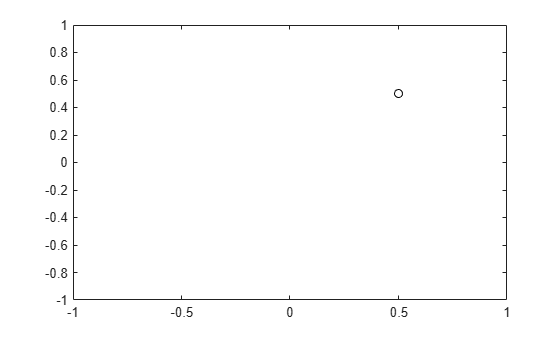# rotatepoint

Quaternion point rotation

Since R2019b

## Syntax

``rotationResult = rotatepoint(quat,cartesianPoints)``

## Description

example

````rotationResult = rotatepoint(quat,cartesianPoints)` rotates the Cartesian points using the quaternion, `quat`. The elements of the quaternion are normalized before use in the rotation.```

## Examples

collapse all

Define a point in three dimensions. The coordinates of a point are always specified in order x, y, z. For convenient visualization, define the point on the x-y plane.

```x = 0.5; y = 0.5; z = 0; plot(x,y,"ko") hold on axis([-1 1 -1 1])```Create a quaternion vector specifying two separate rotations, one to rotate the point 45 and another to rotate the point -90 degrees about the z-axis. Use `rotatepoint` to perform the rotation.

```quat = quaternion([0,0,pi/4; ... 0,0,-pi/2],"euler","XYZ","point"); rotatedPoint = rotatepoint(quat,[x,y,z])```
```rotatedPoint = 2×3 -0.0000 0.7071 0 0.5000 -0.5000 0 ```

Plot the rotated points.

```plot(rotatedPoint(1,1),rotatedPoint(1,2),"bo") plot(rotatedPoint(2,1),rotatedPoint(2,2),"go")```Define two points in three-dimensional space. Define a quaternion to rotate the point by first rotating about the z-axis 30 degrees and then about the new y-axis 45 degrees.

```a = [1,0,0]; b = [0,1,0]; quat = quaternion([30,45,0],"eulerd","ZYX","point");```

Use `rotatepoint` to rotate both points using the quaternion rotation operator. Display the result.

`rP = rotatepoint(quat,[a;b])`
```rP = 2×3 0.6124 0.5000 -0.6124 -0.3536 0.8660 0.3536 ```

Visualize the original orientation and the rotated orientation of the points. Draw lines from the origin to each of the points for visualization purposes.

```plot3(a(1),a(2),a(3),"bo"); hold on grid on axis([-1 1 -1 1 -1 1]) xlabel("x") ylabel("y") zlabel("z") plot3(b(1),b(2),b(3),"ro") plot3(rP(1,1),rP(1,2),rP(1,3),"bd") plot3(rP(2,1),rP(2,2),rP(2,3),"rd") plot3([0;rP(1,1)],[0;rP(1,2)],[0;rP(1,3)],"k") plot3([0;rP(2,1)],[0;rP(2,2)],[0;rP(2,3)],"k") plot3([0;a(1)],[0;a(2)],[0;a(3)],"k") plot3([0;b(1)],[0;b(2)],[0;b(3)],"k")```## Input Arguments

collapse all

Quaternion that defines rotation, specified as a `quaternion` object or a vector of `quaternion` objects. `quat` and `cartesianPoints` must have compatible sizes:

• `length(quat) == size(cartesianPoints,1)`, or

• `length(quat) == 1`, or

• `size(cartesianPoints,1) == 1`

Three-dimensional Cartesian points, specified as a 1-by-3 numeric vector representing a single point or an N-by-3 numeric matrix representing N points. `quat` and `cartesianPoints` must have compatible sizes:

• `length(quat) == size(cartesianPoints,1)` or

• `length(quat) == 1`, or

• `size(cartesianPoints,1) == 1`

Data Types: `single` | `double`

## Output Arguments

collapse all

Rotated Cartesian points defined using the quaternion rotation, returned as a 1-by-3 numeric vector or a numeric matrix.

`rotationResult` is a 1-by-3 vector when `quat` is a scalar `quaternion` object and `cartesianPoints` is a 1-by-3 vector representing a single point. Otherwise, `rotationResult` is an M-by-3 matrix, where M is the maximum of `length(quat)` and `size(cartesianPoints,1)`.

Data Types: `single` | `double`

## Algorithms

Quaternion point rotation rotates a point specified in R3 according to a specified quaternion:

`${L}_{q}\left(u\right)=qu{q}^{*}$`

where q is the quaternion, * represents conjugation, and u is the point to rotate, specified as a quaternion.

For convenience, the `rotatepoint` function takes in a point in R3 and returns a point in R3. Given a function call with some arbitrary quaternion, q = a + bi + cj + dk, and arbitrary coordinate, [x,y,z], for example,

`rereferencedPoint = rotatepoint(q,[x,y,z])`
the `rotatepoint` function performs the following operations:

1. Converts point [x,y,z] to a quaternion:

`${u}_{q}=0+xi+yj+zk$`
2. Normalizes the quaternion, q:

`${q}_{n}=\frac{q}{\sqrt{{a}^{2}+{b}^{2}+{c}^{2}+{d}^{2}}}$`
3. Applies the rotation:

`${v}_{q}=q{u}_{q}{q}^{*}$`
4. Converts the quaternion output, vq, back to R3

## Version History

Introduced in R2019b